# Local uniformizing parameter

local uniformizer, local parameter

A complex variabledefined as a continuous function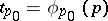of a pointon a Riemann surface, defined everywhere in some neighbourhood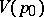of a point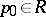and realizing a homeomorphic mapping ofonto the disc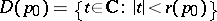, where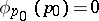. Hereis said to be a distinguished or parametric neighbourhood,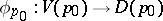a distinguished or parametric mapping, and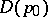a distinguished or parametric disc. Under a parametric mapping any point function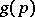, defined in a parametric neighbourhood, goes into a function of the local uniformizing parameter, that is,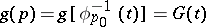. Ifand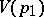are two parametric neighbourhoods such that, and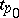and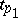are the two corresponding local uniformizing parameters, thenis a univalent holomorphic function on some subdomain ofrealizing a biholomorphic mapping of this subdomain into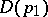.

Ifis the Riemann surface of an analytic function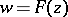and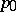is a regular element ofwith projection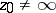, then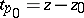;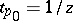for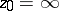. Ifis a singular, or algebraic, element of, corresponding to the branch point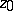of order, then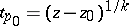forandfor. In a parametric neighbourhood of an elementthe local uniformizing parameteractually realizes a local uniformization, generally speaking, of the many-valued relation, according to the formulas (for example, for):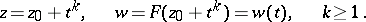In the case whenis a Riemann surface with boundary, for pointsbelonging to the boundary ofthe local uniformizing parametermaps the parametric neighbourhoodonto the half-discIfis a a Riemannian domain over a complex space,, then the local uniformizing parameter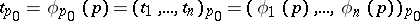realizes a homeomorphic mapping of the parametric neighbourhoodonto the polydisc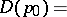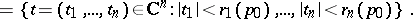If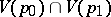is not empty, then the mapping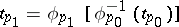biholomorphically maps a certain subdomain ofinto.